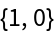#Function Repository Resource:

# CenteredSimplex

Return an origin-centered simplex where the square of all edge lengths is 2

Contributed by: Ed Pegg Jr
 ResourceFunction["CenteredSimplex"][dim] returns an origin-centered simplex with dimension dim.

## Examples

### Basic Examples (3)

A simplex in 3D, otherwise known as a regular tetrahedron:

 In:=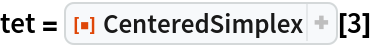Out=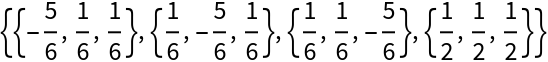The center is the origin and all edge-lengths are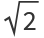:

 In:=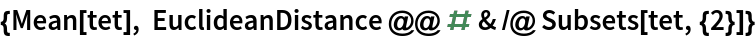Out=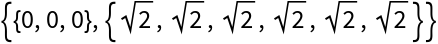Show the simplex:

 In:=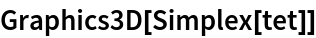Out=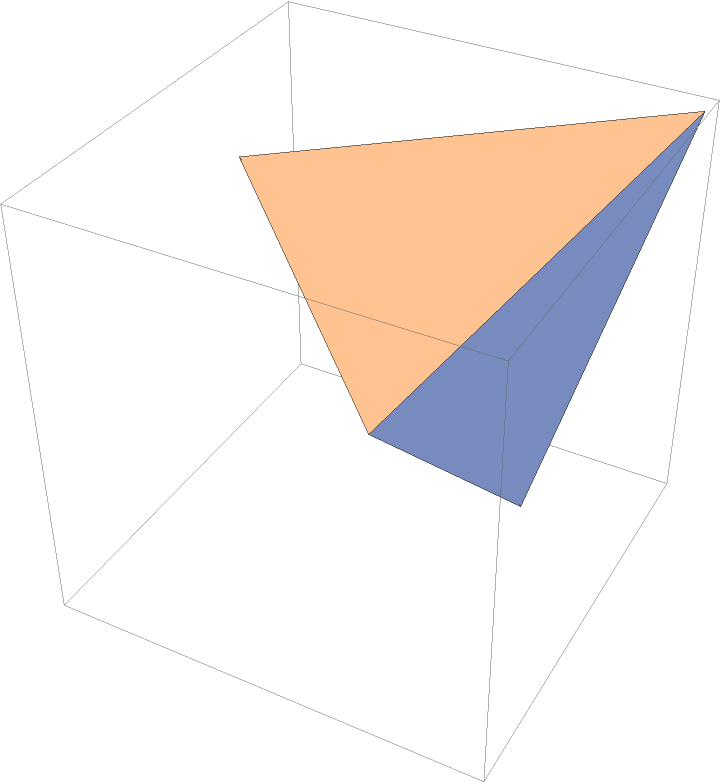### Properties and Relations (2)

The last vertex of a k-dimensional CenteredSimplex output is a vector of length k with each coordinate having value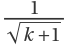:

 In:=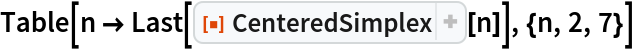Out=The other k vertices are permutations of one another:

 In:=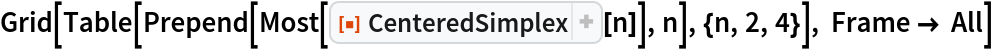Out=Select two random points in 3D space:

 In:=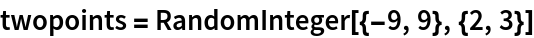Out=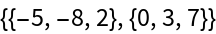We can use the centered simplex system when converting the points to barycentric coordinates, the latter of which can be done using the resource function BarycentricCoordinates:

 In:=Out=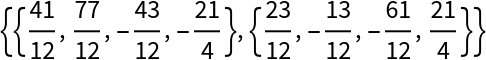When a regular simplex with edge-lengths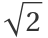is used for barycentric coordinates, the Euclidean distance is preserved:

 In:=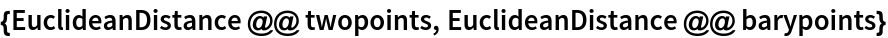Out=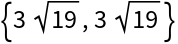### Neat Examples (2)

We can appendto each vertex to get a square matrix:

 In:=Out=This matrix has determinant 1 as well as mutually orthogonal rows and columns:

 In:=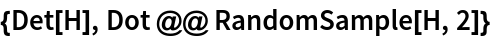Out=